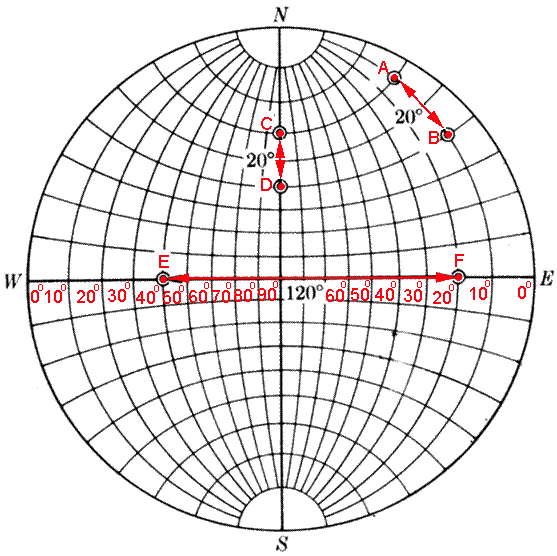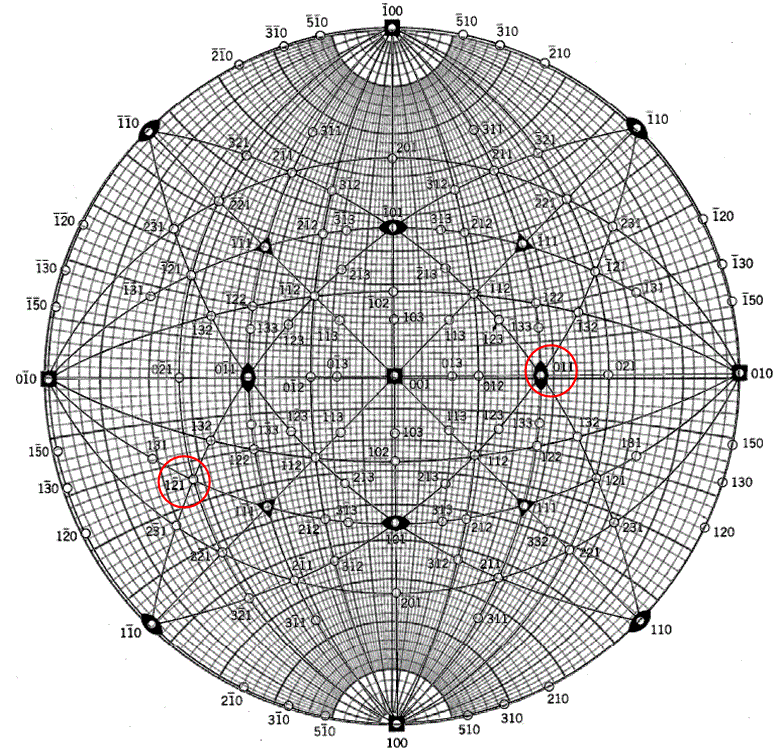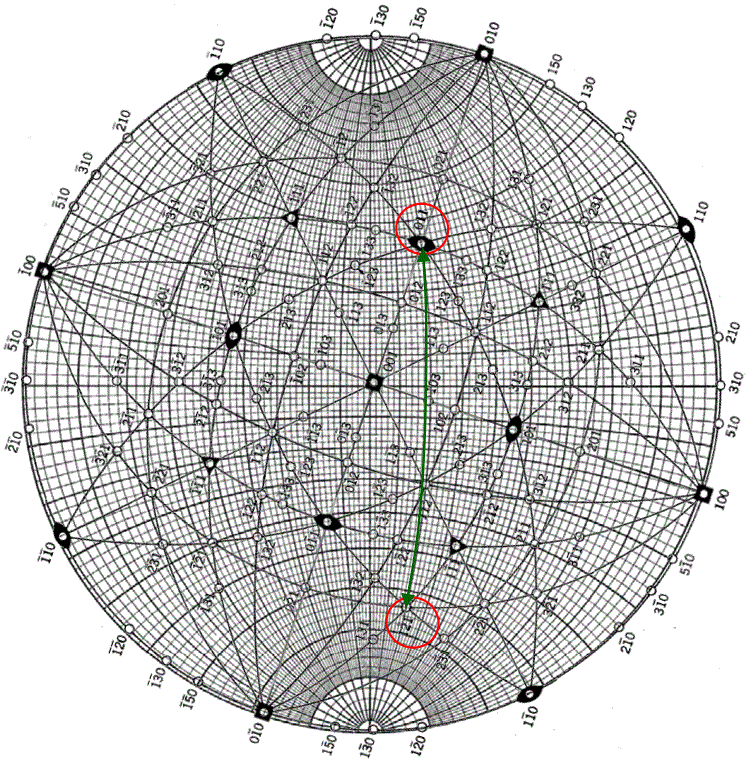Angle between Two Planes/Plane Normals/Poles
Measured by Wulff Net
- Practical Electron Microscopy and Database -
- An Online Book -

http://www.globalsino.com/EM/

This book (Practical Electron Microscopy and Database) is a reference for TEM and SEM students, operators, engineers, technicians, managers, and researchers.

=================================================================================

The angle between any two planes is the angle between their plane normals. They can be measured along a great circle using the Wulff net.

Functions of Wulff Net

To measure the angle between two pole directions
To find the pole of great circle
To find the great circle corresponding to a pole
To find the angle between two planes
To rotate projection around an axis lying in the plane of projection.

Figure 4118a shows the angles between different pairs of poles (representing planes in crystallography) measured by Wulff Net. For instance, the angles between the pairs of poles (A and B, C and D, and E and F poles) are 20°, 20°, and 120°, respectively.Figure 4118a. Angles between different pairs of poles (representing planes in crystallography) measured by Wulff Net. Here, E stands for east, S for south, W for west, and N for north. Latitude measures north-south position and longitude measures east-west.

Example of finding the angle between two planes with stereographic projection:
Case i) Assuming the poles A and B are on the edge of the stereographic projection as shown in Figure 4118b.
Step i.a) Overlay the Wulff net in any orientation.
Step i.b) Quantify the angle between the two planes by counting the tick marks on the edge.Figure 4118b. Poles on the edge of the stereographic projection.

Case ii) To find the angle between the poles 1-21 and 011 in stereographic projection for a cubic film with  normal as shown in Figure 4118c.Figure 4118c. Poles 1-21 and 011 (marked with red circles) in stereographic projection for a cubic film with  normal.

Step ii.a) Overlay Wulff net with the stereographic projection (in Figure 4118c) on top as shown in Figure 4118d.Figure 4118d. Overlaid Wulff net with the stereographic projection in Figure 4118c.

Step ii.b) Rotate the stereographic projection about the center until both poles 1-21 and 011 are intersected by a common line of longitude, i.e. on the same great circle as shown in Figure 4118e.Figure 4118e. Both poles 1-21 and 011 on the same great circle.

Step ii.c) Count the latitude ticks along the green arrowed line of longitude to obtain the angle, which is ~107°. The measured value can be verified by theoretically calculating the angle between the two normals (poles) for cubic systems (see page3086):-------------------- [4118a]---------- [4118b]
Therefore, φ = 106.8° = ~107°, and thus the measurement is correct. Note that the lines of latitude will not work as they are not great circles of the sphere. Therefore, do not put both poles 1-21 and 011 intersected by a common line of latitude. Furthermore, the method described above is applicable to any two arbitrary poles.

=================================================================================

The book author (Yougui Liao) welcomes your comments, suggestions, and corrections, please click here for submission. If you let book author know once you have cited this book, the brief information of your publication will appear on the “Times Cited” page.